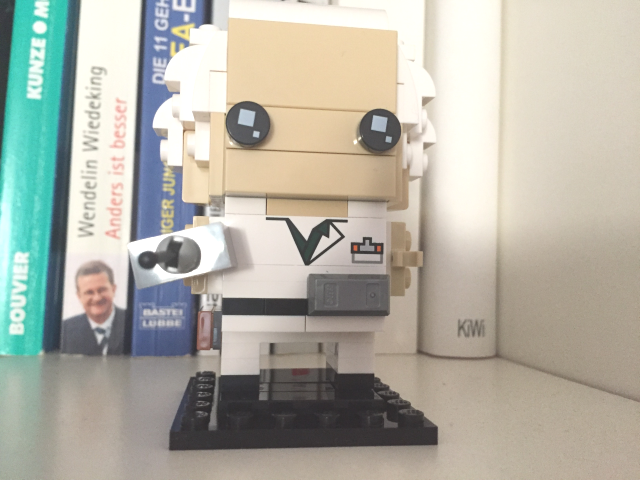# Intro to OpenCV with Python

To work with OpenCV from python, you need to install it first:

`pip install opencv-python`

After we import cv2 we can directly work with images like so:

```import cv2
```

For showing the image, it is recommended to use matplotlib

```import matplotlib.pyplot as plt
img = cv2.cvtColor(img, cv2.COLOR_BGR2RGB)
plt.imshow(img)
plt.show(```

OpenCV stores images internally in the BGR format – blue – green – red so we have to convert to RGB before displaying them.

## Image Shape

We have now an image object which can already tell us more about the image itself:

```print(img.shape)
(205, 236, 3)```

The tuple shows us the number of (rows, columns, channels)

## Manipulate brightness

```import numpy as np
brightness = np.zeros(img.shape, dtype="uint8") + 30

Manipulating brightness works like this: you need a numpy array with the size of the image, add your brightness and add the brightness array to the original image.

##```noise = np.random.randint(0, 100, size=img.shape, dtype="uint8")## Smoothing

Smoothing is reducing the noise of an image by adding a Gaussian blur:

`img = cv2.GaussianBlur(img, ksize=(31, 31), sigmaX=5)`

## Gray-scale conversion

Last but not least we can convert an image to a gray scale. Beware that for showing / storing the image you need to add the flag cmap=”gray”

```img = cv2.cvtColor(img, cv2.COLOR_BGR2GRAY)
plt.imshow(img, cmap='gray')```Have fun fiddling around with OpenCV!